# 3D Shapes Worksheet Vertices, Edges, Faces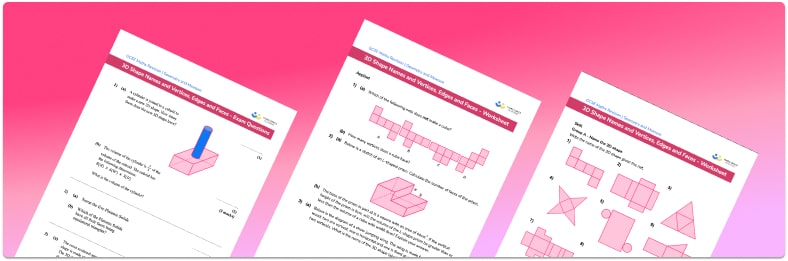• Section 1 of the 3D shapes, vertices, edges, faces worksheet contains 36 skills-based 3D shapes, vertices, edges, faces questions, in 3 groups to support differentiation
• Section 2 contains 4 applied 3D shapes, vertices, edges, faces questions with a mix of worded problems and deeper problem solving questions
• Section 3 contains 4 foundation and higher level GCSE exam style 3D shapes, vertices, edges, faces questions
• Answers and a mark scheme for all 3D shapes, vertices, edges, faces questions are provided
• Questions follow variation theory with plenty of opportunities for students to work independently at their own level
• All questions created by fully qualified expert secondary maths teachers
• Suitable for GCSE maths revision for AQA, OCR and Edexcel exam boards
• There are other free printable worksheets covering geometric shapes, including properties of 2D shapes worksheets.

• This field is for validation purposes and should be left unchanged.

You can unsubscribe at any time (each email we send will contain an easy way to unsubscribe). To find out more about how we use your data, see our privacy policy.

### 3D shapes, vertices, edges, faces at a glance

A three-dimensional shape (3D shape) has three dimensions, length, width and height. Students are expected to recognise solid shapes such as cubes, cuboids (or rectangular prisms) and triangular prisms. We live in a three-dimensional world, so students should be familiar with the real life examples of different 3D shapes around them.

3D shape properties include the number of faces, edges and vertices of 3d shapes. A face is a flat or curved surface. An edge is where two faces meet, and a vertex is a corner where edges meet. If the faces of a 3-dimensional shape are all polygons, it is called a polyhedron. If all faces are the same regular polygon, it is called a regular polyhedron. An example of a regular polyhedron is a tetrahedron which has four equilateral triangular faces.

Euler’s formula links the number of faces, vertices and edges. It states that for any polyhedron, the number of faces plus the number of vertices minus the number of edges will always be 2. This is not examined at GCSE but can be a good enrichment task when studying properties of 3D shapes and is a great introduction to a very famous mathematician.

A prism is a geometric shape that has a constant cross-section. If we cut a prism parallel to its end, we reveal an identical 2D shape. An example of a prism is a hexagonal prism; it consists of two hexagonal faces at either end, and six rectangular faces joining the hexagons. If we cut this prism through the rectangular faces, the 2D shape revealed inside would be a hexagon.

There are different 3D shapes activities students can do to learn properties of 3D shapes. For example, it can be useful for students to create a set of revision flashcards with the name, diagram and properties of each 3D shape. Creating a 3D paper model or a net or using real life examples can help make 3D shapes interactive and allow students to identify and count faces, edges and vertices on the shape.

Looking forward, students can then progress to additional geometry worksheets, for example an angles in polygons printable worksheet or how to calculate volume printable worksheet.For more teaching and learning support on Geometry our GCSE maths lessons provide step by step support for all GCSE maths concepts.

## Related worksheets

Congruent Triangles Worksheet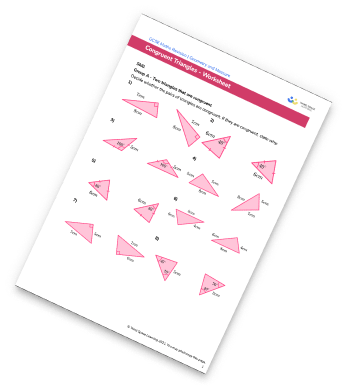Volume Worksheet – How To Calculate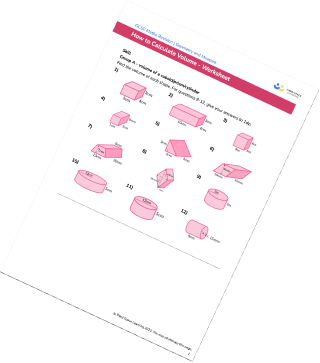Transformations Worksheets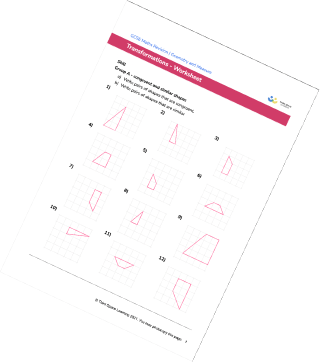Translating Shapes Worksheet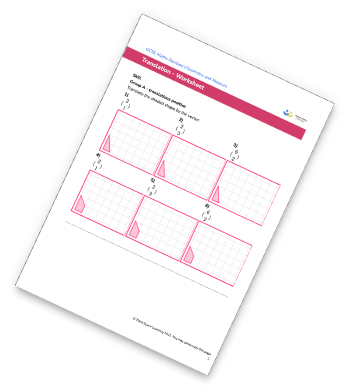## Do you have KS4 students who need more focused attention to succeed at GCSE?There will be students in your class who require individual attention to help them succeed in their maths GCSEs. In a class of 30, it’s not always easy to provide.

Help your students feel confident with exam-style questions and the strategies they’ll need to answer them correctly with our dedicated GCSE maths revision programme.

Lessons are selected to provide support where each student needs it most, and specially-trained GCSE maths tutors adapt the pitch and pace of each lesson. This ensures a personalised revision programme that raises grades and boosts confidence.

Find out more